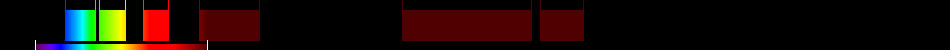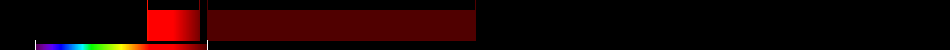# Information for Sensor and Index

## Formula

General Formula $\frac{{\mathrm{NIR}}}{{\mathrm{RED}}+\frac{{b}}{{a}}}$ $\frac{{4}}{{3}+\frac{{b}}{{a}}}$ Automatic

## Sensor

Name Resource21 Resource21 6 450-1650 10-100 98 Resource21 1999-00-00 Off-Nadir Repeat Cycle: Twice in 25 min (with 4 satellites) yes

## Index

Name Soil-adjusted vegetation index 2 SAVI2 $\frac{{\mathrm{NIR}}}{{\mathrm{RED}}+\frac{{b}}{{a}}}$ The actual gain (a) and intercept (b) values of the soil line rather than assuming them to be 1 and 0, respectively 640:760,780:1400 Original formula

## References